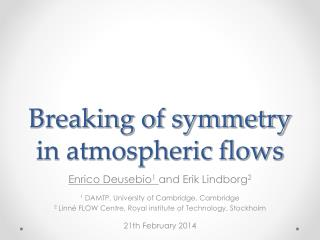DownloadDownload PresentationBreaking of symmetry in atmospheric flows

# Breaking of symmetry in atmospheric flows

Télécharger la présentation## Breaking of symmetry in atmospheric flows

- - - - - - - - - - - - - - - - - - - - - - - - - - - E N D - - - - - - - - - - - - - - - - - - - - - - - - - - -
##### Presentation Transcript

1. Breaking of symmetry in atmospheric flows Enrico Deusebio1and Erik Lindborg2 1 DAMTP, University of Cambridge, Cambridge 2 Linné FLOW Centre, Royal institute of Technology, Stockholm 21th February 2014

2. Symmetry and asymmetry Symmetry often observed in nature and generally thought as a sign of perfection and beauty

3. Symmetry and asymmetry Symmetry often observed in nature and generally thought as a sign of perfection and beauty Used by mathematicians to simplify their systems

4. Symmetry and asymmetry Symmetry often observed in nature and generally thought as a sign of perfection and beauty Used by mathematicians to simplify their systems Also used in numerical simulations in order to reduce the computational cost

5. Asymmetry in atmospheric flows Are atmospheric flows symmetric?

6. Asymmetry in atmospheric flows Are atmospheric flows symmetric?

7. Asymmetry in atmospheric flows Are atmospheric flows symmetric?

8. Asymmetry in atmospheric flows Are atmospheric flows symmetric?

9. Asymmetry in atmospheric flows Are atmospheric flows symmetric? WHY? Coriolis terms (not symmetry invariant) Can this be observed at small scales?

10. Helicity (Moffatt & Tsinober, 1992)

11. Helicity Velocity (Moffatt & Tsinober, 1992)

12. Helicity Vorticity (Moffatt & Tsinober, 1992)

13. Helicity (Moffatt & Tsinober, 1992) Invariant of the Euler equations, thus conserved apart from viscous effects

14. Helicity (Moffatt & Tsinober, 1992) Invariant of the Euler equations, thus conserved apart from viscous effects AS ENERGY

15. Helicity (Moffatt & Tsinober, 1992) Invariant of the Euler equations, thus conserved apart from viscous effects AS ENERGY Both positive and negative values can be attained

16. Helicity (Moffatt & Tsinober, 1992) Invariant of the Euler equations, thus conserved apart from viscous effects AS ENERGY Both positive and negative values can be attained DIFFERENTLY FROM ENERGY

17. Turbulent Ekman boundary layers Recent numerical simulations of turbulent Ekman layers up to Standard numerics using pseudo-spectral codes

18. Budget equations

19. Budget equations

20. Budget equations

21. Budget equations Energy dissipation Energy injection

22. Budget equations Energy dissipation Energy injection

23. Budget equations Energy dissipation Energy injection Helicity dissipation Helicity injection

24. Budget equations Mean field helicity Turbulent helicity Production Transport Pressure Dissipation Rotation

25. Budget equations Mean field helicity Turbulent helicity Production Transport Pressure Dissipation Rotation Conversion from mean to turbulent helicity

26. Budget equations Mean field helicity Turbulent helicity Production Transport Pressure Dissipation Rotation Viscous dissipation of helicity

27. Budget equations Mean field helicity Turbulent helicity Production Transport Pressure Dissipation Rotation Transport of helicity from one place to another

28. Budget equations Mean field helicity Turbulent helicity Production Transport Pressure Dissipation Rotation Transport of helicity due to the pressure terms

29. Budget equations Mean field helicity Turbulent helicity Production Transport Pressure Dissipation Rotation Transport of helicity due to rotation

30. Budget equations Production Transport Pressure Dissipation Rotation

31. Budget equations Production Transport Pressure Dissipation Rotation

32. The helicity cascade Injection of helicity Helicity dissipation

33. Asymmetry in atmospheric flows Are atmospheric flows symmetric? WHY? Coriolis terms (not symmetry invariant) Can this be observed at small scales?

34. Asymmetry in atmospheric flows Are atmospheric flows symmetric? WHY? Coriolis terms (not symmetry invariant) Can this be observed at small scales? YES, WE CAN!

35. Thank you for the attention!

36. Towards the measurements…

37. Ekman boundary layers Balance between viscous and Coriolis terms Geostrophic Wind far from surface

38. Budget equations In steady conditions the total helicity is determined by a balance between the injection (due to the pressure gradient) and the viscous destruction Total helicity injection

39. Turbulent Ekman boundary layers Recent numerical simulations of turbulent Ekman layers up to Standard numerics using pseudo-spectral codes